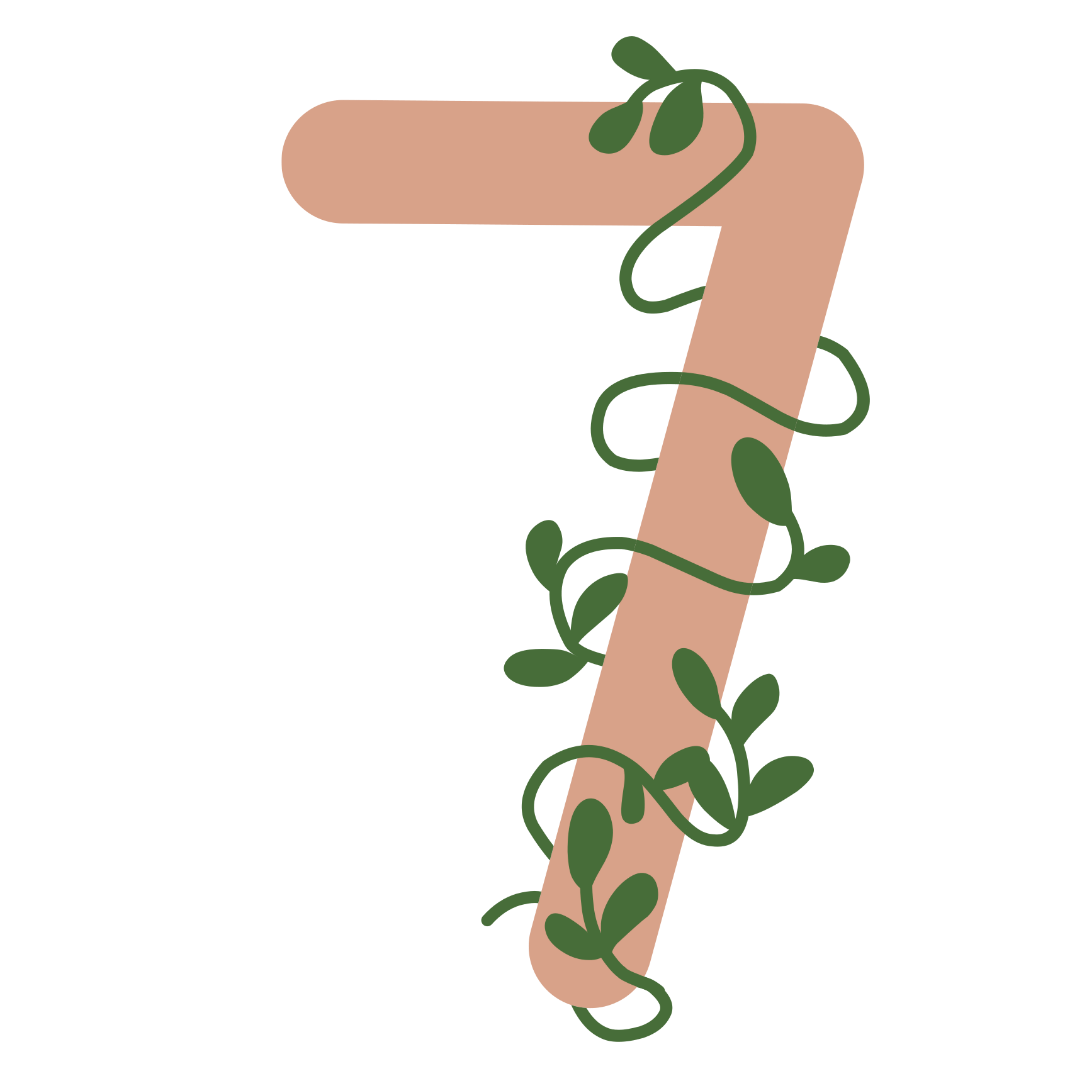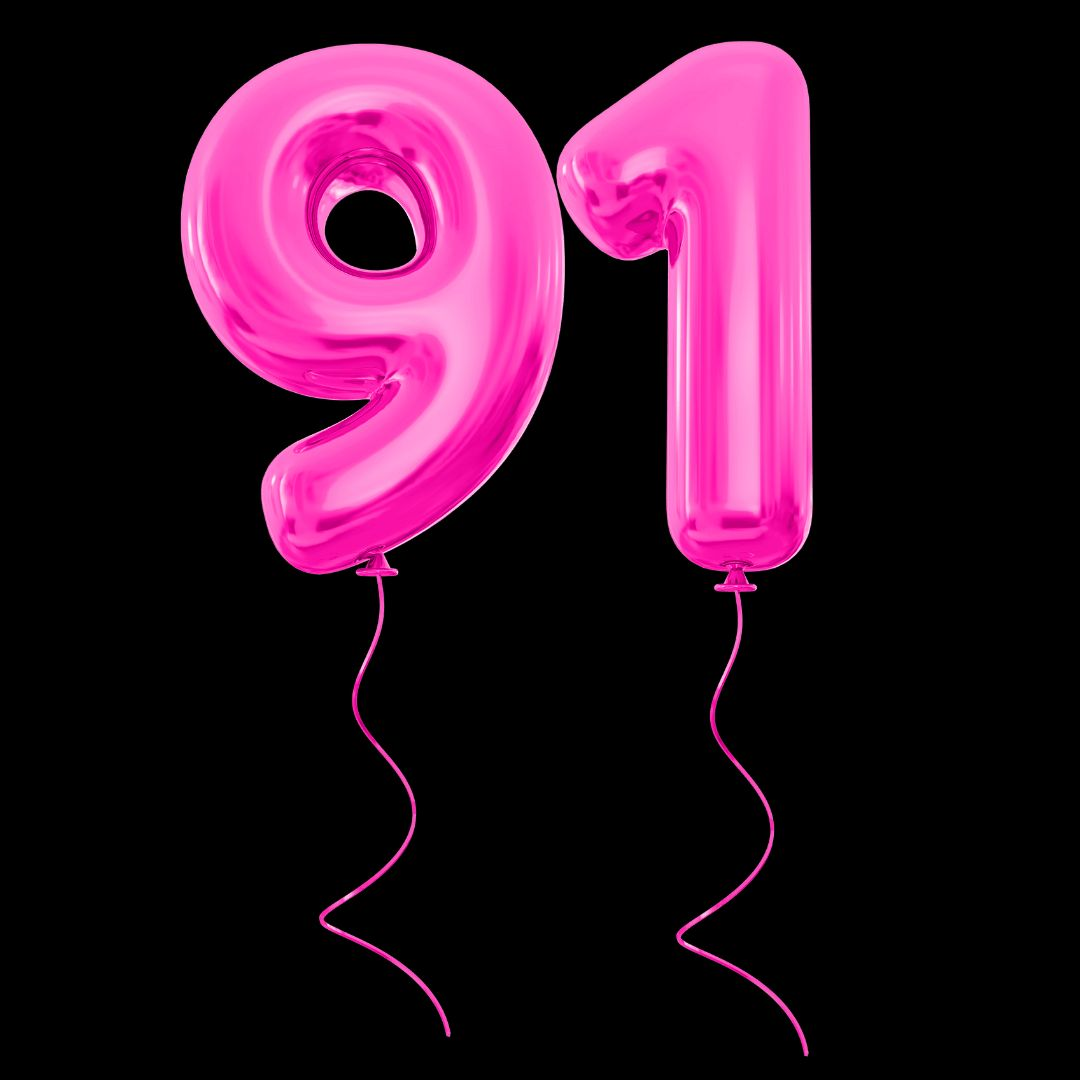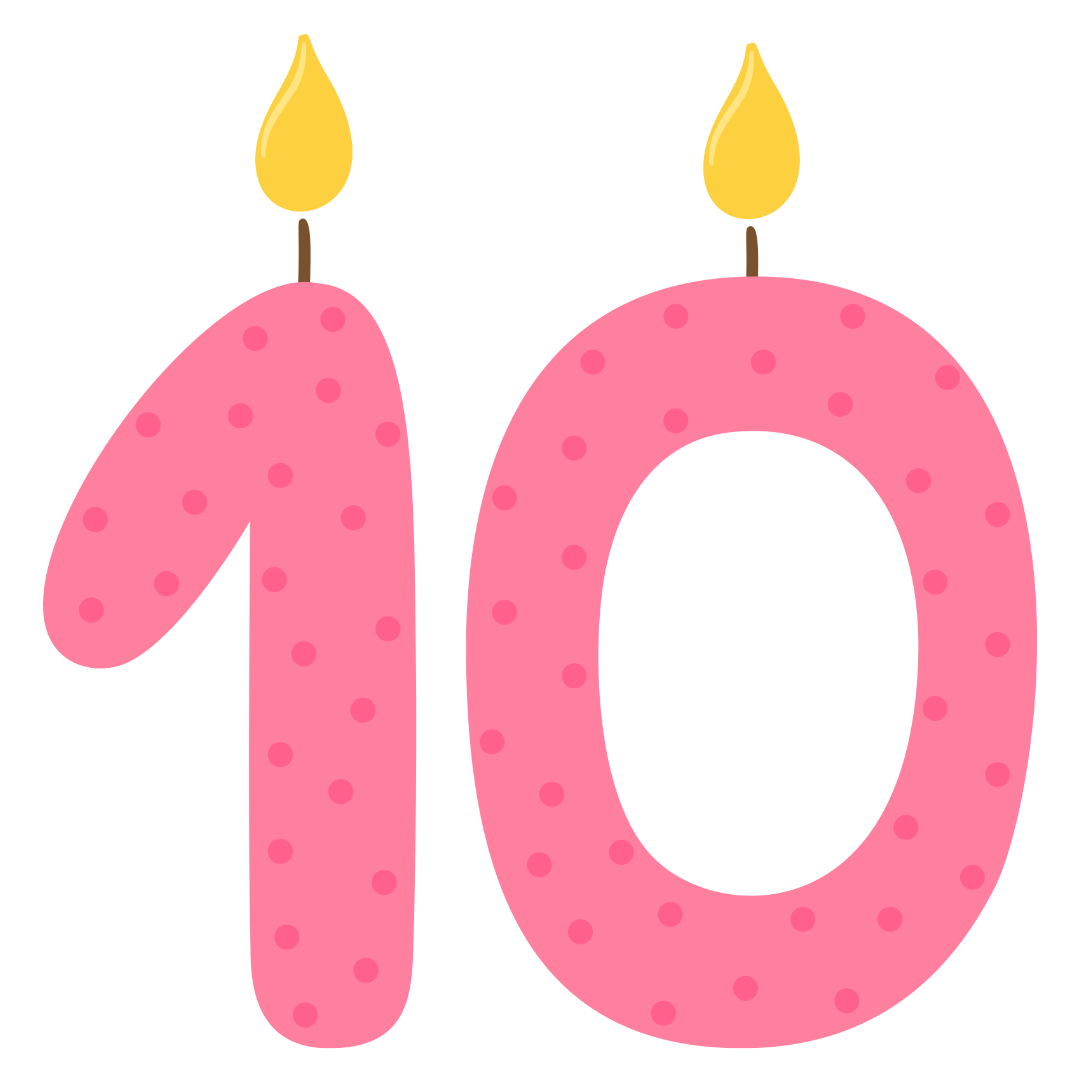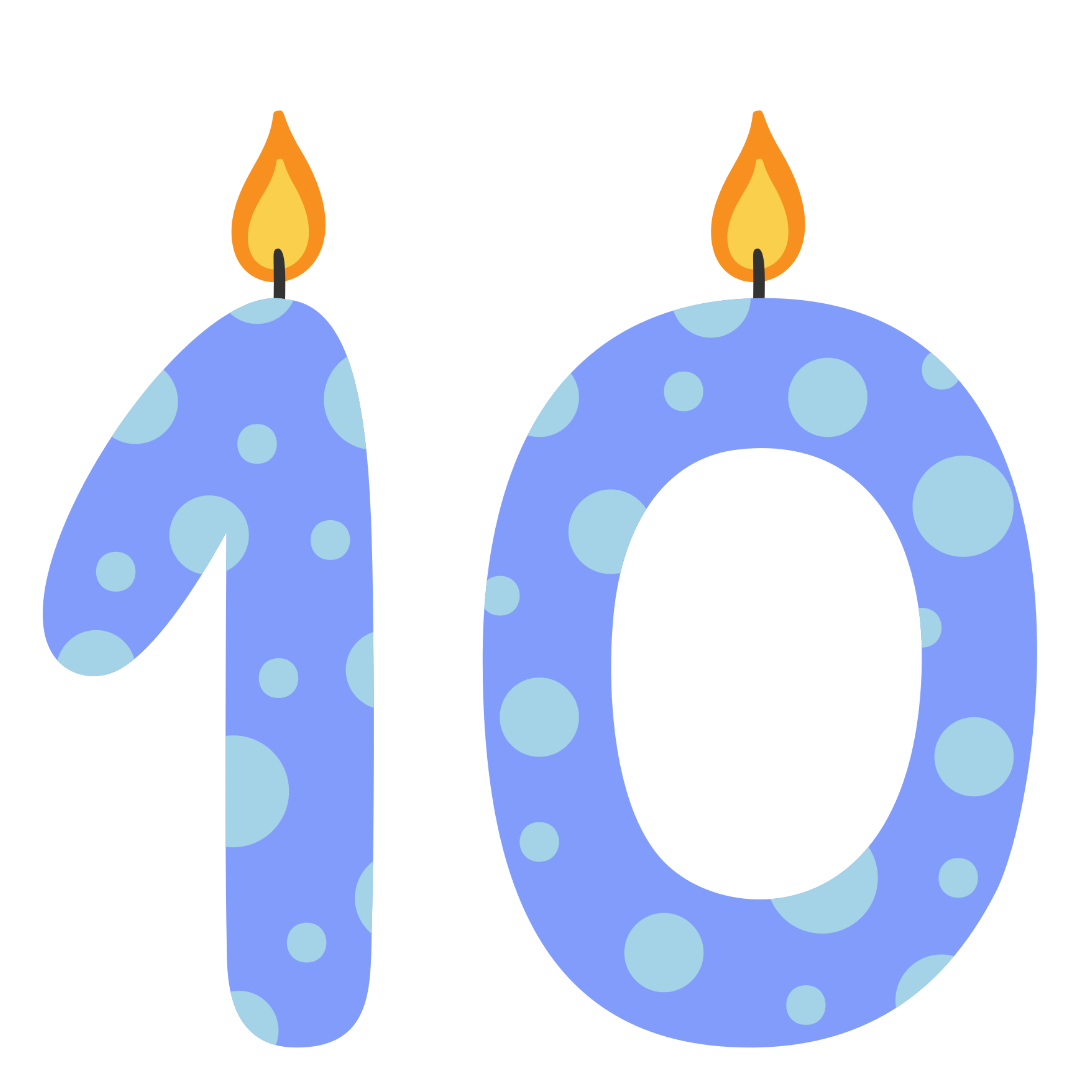Courses
Courses for Kids
Free study material
Free LIVE classes
More

# Maths Worksheet for Kids- Greater Than and Less ThanLIVE
Join Vedantu’s FREE Mastercalss

## Maths Worksheet for Kids| Greater Than and Less Than - Download Free PDF with Solutions

Greater Than and Less Than is one of the most important topics for students of Class KG-3. The topic introduces students to a variety of numbers, among which they have to choose the numbers that are larger than the others. This type of elementary knowledge is essential for students in their higher studies. Students can download Greater Than and Less Than worksheets from Vedantu and solve the questions to gain more knowledge about the topic.

The experts at Vedantu have curated the worksheets for Greater Than and Less Than to help students compare different numbers and place them based on their order. Students will be introduced to a new concept and can answer questions based on the concepts to boost their abilities. Such questions and worksheets are designed to improve their logical and numerical sense. Download the More and Less worksheets from Vedantu and start practising the art of numbers.

### Did You Know?

We know that 5 is larger than 4, and 2 is larger than 1. But do you know that -1 is larger than -2 and -9 is larger than -10? This is because the numbers with the ‘-‘sign belong to the negative side of the number line. This means the numbers with less values are larger than the ones that have more value, accompanied when with a negative sign.

Last updated date: 03rd Oct 2023
Total views: 22.2k
Views today: 1.22k

## Access Worksheet for Maths KG-2 Greater Than and Less Than

Greater than and less than means a number which is bigger or smaller than the given number. For example, 5 is greater than 4 and 6 is lesser than 5. If Raj is 10 years old and his father is 47 years old, than his father is older than him.

### QUESTIONS:

1. Write the sign used for bigger than.

2. Write the sign used for smaller than.

3. Mention <,> or = in the blanks.

1. 5 __ 10

2. 7 __ 4

3. 9 __ 5

4. 10 __10

4. If Sneha is 9 years old and her sister is 10 years old, then who is younger, and by what difference?

5. Match the following images with their respective numbers.

 Column A Column BNineSevenFive

6. True or false.

1. 9 is smaller than 10.

2. 11 is bigger than 14.

3. 10 is equal to 10.

7. In the given images put a sign of <,> or = .

1.an image of 91an image of 100

1.an image of 10

____an image of 10.

1.an image of 12

_____an image of 10

8. Write 3 consecutive less numbers than the following numbers.

1. 11

2. 9

3. 8

9. Write 3 consecutive greater number than the following numbers.

1. 12

2. 6

3. 9

4. 11

5. 13

9. By what difference Rajesh is older than his sister, if he is 19 years old and his sister is 15 years old.

10. If you have 100 rupees and you want book worth 120 rupees than you have less or more money to purchase the books.

11. Raj have 10 flowers and Riya have 15 flowers how many more flowers does Riya have?

12. Write a number smaller than 3.

13. If A is 3 years younger than B and B is 14 years old what will be the age of A?

14. If Z is 20 years old and A is 5 years younger and C is 10 years older than him what will be the age of A and C?

1. Sign we use for bigger than is ‘<’.

2. Sign we use for smaller than is ‘>’.

3. Putting <,> or =

1. 5 < 10

2. 7 > 4

3. 9 >

4. 10 =10

4. Sneha’s age is 9 and her sister is 10,

So, 10-9 is 1

Sneha is 1 year younger and her sister is 1 year older than her.

5.

 Column A Column BFiveNineSeven

6.

(a) true

(b) false

(c) true

7. Putting the sign <,> or =

1.image- an image of ninety-onean image of 100

1.an image of 10.an image of 10

1.an image of 12an image of 10

8. Consecutive means serial vise

1. 11 = 10, 9, 8

2. 9 = 8, 7, 6

3. 8 = 7, 6, 5

9. Consecutive greater number

1. 12 = 13, 14, 15

2. 6 = 7, 8, 9

3. 9 = 10, 11, 12

4. 11 = 12, 13, 14

5. 13 = 14, 15, 16

10. Rajesh is 19 and his sister is 15

15-19= 4, thus Rajesh is 4 years older than his sister.

11. Books cost is 120 and I have 100

120-100 = 20, thus I have 20 rupees less than the cost of books.

12. Raj have 10 flowers and Riya have 15

So, 15-10=5, thus Riya has 5 more flowers than Raj.

13. A number smaller than 3 is 2.

14. A is 3 years younger than B and B is 14 years old,

14-3=11, thus A is 11 years old.

15.  Z is 20 years old, A is 5 years younger than Z and C is 10 years older than Z,

20-5 =15, A is 15 years old.

20+10 =30, C is 30 years old.

## Importance of Greater Than Less Than Worksheets

The students of Class KG-3 will be introduced to the concepts of Greater Than and Less Than in their syllabus. There are different worksheets on Vedantu that will help the children learn and properly compare the numbers. These worksheets are also a great way to provide a proper introduction to the Less Than Greater Than Symbol. These sheets have been arranged in the order of their difficulty and students can choose from the easiest sheets first while moving on to the more challenging sheets later on.

In the beginning of the worksheets, students can shade the larger of the two numbers provided to them. Moving further along the sheets, students will be able to perform addition and subtraction to find the larger numbers. This is something that is going to help them understand the questions properly. Download the Greater Than Less Than Worksheets to learn easily and improve your concepts.

### Benefits of Great Than Less Than Worksheets With Answers

• Students can download the greater than less than worksheets and start practising to be completely prepared for their exams. They can gain better knowledge of the concepts.

• The worksheets have been designed by an expert panel of academicians at Vedantu in order to help the students properly grasp the concepts. They can download the worksheets and practice.

• Students will be able to download the worksheets for free and then access them offline anytime. There are different types of worksheets that contain fun activities for children to make the learning process easier.

• Students can solve the questions and improve their addition, subtraction, and other mathematical skills in the best way. There are solutions provided in the worksheets that students can refer to in order to get a good understanding.

If you want to improve your knowledge about numbers, download the Greater Than Less Than Worksheets with answers from Vedantu. Here you will find the solved questions to refer to and learn things easily.

## FAQs on Maths Worksheet for Kids- Greater Than and Less Than

1. Where can I find Greater Than and Less Than Worksheets?

You can download the Greater Than and Less Than worksheets from the official website of Vedantu. These worksheets are grouped on the basis of their difficulty levels. So, students can get proper practice and move on to different levels with time.

2. Which number is greater between 32 and 57?

57 is the greater number between the two.